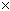SEARCH HOMEMath Central Quandaries & QueriesQuestion from Cynthia, a student: Hi is (x^6)(x^4)1/2 = 5 And How does 1/2(3.14)(4.1) =26.3917Cynthia, we have two responses for you:

Hi Cynthia,

I am not sure what you are asking with the first question. Are you to solve for x?

For the second question 1/2(3.14)(4.1) is not 26.3917. Try 1/2(3.14)(4.1)^2

Penny

Hi Cynthia.

For your first question, did you mean to ask if ( (x6 )(x4 ) )½ = x5 ?

If so, then the answer is yes, because when you first combine (x6 )(x4 ) you use the exponent rule of multiplication: add the exponents, so this is (x10). Then when you take this to the exponent of ½, you use the exponent rule of exponents: multiply the exponents. Since 10½ = 5 , then (x10)½ = x5.

Stephen La Rocque.>Math Central is supported by the University of Regina and The Pacific Institute for the Mathematical Sciences.